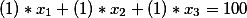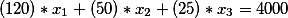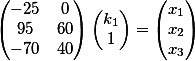# System of linear equations

PsychonautQQ

## Homework Statement

How do I solve the following system?
x+y+z = 100
120x + 50y + 25z = 4000

where x, y, and z must all be at least one and are all integers. There will be more than one solution right

none.

## The Attempt at a Solution

I solved for z in terms of the other two variables and got z must be between 43 and 84. I choose p = 50 and then tried to solve for c but didn't get an integer. Is there a more systematic way to approach this question?

Homework Helper
Gold Member
Systematic way? I don't know the answer. I also don't know whether it really helps but you would at least show you had done something if you divided the second equation by 5. You can in any case get three (<) inequalities for x, y and z without solving any equations. And you caN get inequalities for x and y in the same way you did for z. Then you could see whether that limits the answer enough to be able to solve efficiently. It's obvious isn't it that you can always solve it inefficiently? In fact once you have solved them why don't you... think about everything you know about linear equations?

Last edited:
PsychonautQQ
How do i gaurentee that my answer will be an integer when I select a value for one of my variable within the bounds? seems like ALOT of work/luck to finally come to a proper answer.

Homework Helper
Gold Member
How do i gaurentee that my answer will be an integer when I select a value for one of my variable within the bounds? seems like ALOT of work/luck to finally come to a proper answer.

Well I don't call what I suggested a lot of work and sometimes if you can't foresee a calculation right to the end you just have to see what it is that you can calculate, do it, and then ponder what you might make of the greater information you then have. And even if you can't in the end, you get some credit from teachers, examiners and us.

•PsychonautQQ
Homework Helper
Dearly Missed

## Homework Statement

How do I solve the following system?
x+y+z = 100
120x + 50y + 25z = 4000

where x, y, and z must all be at least one and are all integers. There will be more than one solution right

none.

## The Attempt at a Solution

I solved for z in terms of the other two variables and got z must be between 43 and 84. I choose p = 50 and then tried to solve for c but didn't get an integer. Is there a more systematic way to approach this question?

A better way is to solve for two variables in terms of a third, then just "scan" the possible values of that third variable to see what the other two become. It makes sense to solve for y and z in terms of x, since x is the most restricted variable: we must have x <= 4000/120 = 33.33333 .., so 1 <= x <= 33 for integer-restricted x. If you actually carry out the solution you will be able to see that x is much more restricted than indicated above: requiring that y,z be positive integers reduces the number of possible x values to a small number of possibilities.

•PsychonautQQ
Homework Helper
You can use divisibility: Eliminating z, for example, you get the equation 19x +5y=300 at the end. Isolating x, ##x=\frac {5(60-y)}{19}##. So 60-y has to be divisible by 19 and 0<y<60.

•PsychonautQQ
Homework Helper
From x+y+z = 100, we have z= 100- x- y. Putting that into 120x + 50y + 25z = 4000, 120x+ 50y+ 25(100- x- y)= 120x+ 50y+ 2500- 25x- 25y= 95x+ 25y+ 2500= 4000 or 95x+ 25y= 1500. Divide by 5: 19x+ 5y= 300. That is a "Diophantine equation" and there is a fairly standard way of solving such equations. 5 divides into 19 3 times with remainder 4: 19- (3)5= 4. 4 divides into 5 once with remainder 1: 5- 4= 1. Replace that "4" with 19- (3)5: 5- (19- (3)5)= 4(5)- 1(19)= 1. Multiply by 300: 1200(5)- 300(19)= 300. So one solution to 19x+ 5y= 300 is x= -300, y= 1200. But it is easy to see that x= -300+ 5k, y= 1200- 19k is also a solution for any k: 19(-300+ 5k)+ 5(1200- 19k)= -300(19)+ 1200(5)+ 19(5k)- 5(19k) and the "k" terms cancel.

PsychonautQQ
You can use divisibility: Eliminating z, for example, you get the equation 19x +5y=300 at the end. Isolating x, ##x=\frac {5(60-y)}{19}##. So 60-y has to be divisible by 19 and 0<y<60.

Isn't it possible that 5*(60-y) to be divisible by 19 but not 60-y? I know your statement would be true if 60-y was guaranteed to be a prime, but it's not.

Homework Helper
19 is prime! 5 certainly is not divisible by 19 so 60- y must be. (If 60- y is divisible by 19 either it is 19 or it is not prime!)

•PsychonautQQ
Homework Helper
Isn't it possible that 5*(60-y) to be divisible by 19 but not 60-y? I know your statement would be true if 60-y was guaranteed to be a prime, but it's not.
5 is not divisible by 19 but 5K is. What do you know about K then? :)

•PsychonautQQ
PsychonautQQ
ahhh gee wilikers y'er all sho shmart

Homework Helper

•PsychonautQQ
PsychonautQQ
You are intelligent!

Homework Helper
Just a little slurring. PsychonautQQ has probably been drinking too much.

DimsVS
PsychonautQQ said:
1.Homework Statement
How do I solve the following system?
x+y+z = 100
120x + 50y + 25z = 4000

where x, y, and z must all be at least one and are all integers. There will be more than one solution right
IfthenLast edited by a moderator: